# OLAP Cube and Operations

In this tutorial, we are going to learn about the OLAP Cube and Operations on OLAP Cube in OLAP (Online Analytical Processing). By Harshita Jain Last updated : April 17, 2023

## Introduction

In the previous article of OLAP, we have seen various applications of OLAP, Various types of OLAP, advantages, and disadvantages of OLAP. In this article, we will learn about the,

1. OLAP Cube
2. OLAP Cube Operations

## 1. OLAP Cube

An OLAP cube may be a multi-dimensional array of knowledge. The online analytical process (OLAP) may be a computer-based technique of analyzing knowledge to appear for insights. The term cube here refers to a multi-dimensional dataset, that is additionally known as a Hypercube if the quantity of dimensions is larger than three. A cube is also thought of as a multi-dimensional generalization of a two- or three-dimensional program. For instance, a corporation may like to summarize monetary knowledge by product, by time-period, and by the town to check actual and budget expenses. Product, time, cities and situation (actual and budget) are the data's dimensions as shown in the figure. A cube isn't a "cube" within the strict mathematical sense, as all the edges aren't essentially equal.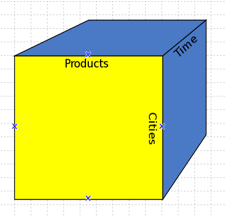Fig 1: OLAP Cube

## 2. OLAP Cube Operations

Since OLAP servers work on data of multidimensional view, we are going to discuss OLAP operations in multidimensional information.

Here is the list of OLAP operations,

• Roll-up
• Drill-down
• Slice
• Dice
• Pivot (rotate)

### 2.1. ROLL UP

Roll-up is performed by rising up a planning hierarchy for the dimension location. Initially the conception hierarchy was "street < town < province < country". On rolling up, aggregation of data is done by ascending the hierarchy of location from the level of street to the level of the country. A roll-up involves summarizing the information on a dimension. The summarization rule may be Associate in Nursing mixture operate, like computing totals on a hierarchy or applying a collection of formulas like "profit = sales - expenses". General aggregation functions are also pricey to cipher once rolling up: if they can not be determined from the cells of the cube, they need to be computed from the bottom information, either computing them on-line (slow) or precomputing them for attainable rollouts (large space). Aggregation functions that will be determined from the cells square measure referred to as complex aggregation functions, and permit economical computation.

For example, it is easy to calculate COUNT, MAX, MIN, and SUM in OLAP, as this can be computed for each cell of the OLAP cube and then rolled up, since on overall sum (or count, etc.) is the sum of sub-sums, but it is difficult to support MEDIAN, as that must be computed for every view separately: the median of a collection isn't the median of medians of subsets.

Let us understand it with a diagrammatic flow,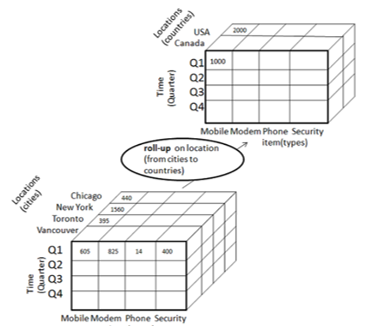Fig 2.1 : Roll up Operation

### 2.2. DRILL DOWN

Drill-down is performed by stepping down the hierarchy for the dimension time. Initially, the conception hierarchy was "day < month < quarter < year." On drilling down, the dimension of time exists in descended from i.e. from the extent of the quarter to the extent of the month. When drill-down is performed, one or additional dimensions from the information cube are supplemental. It navigates knowledge from less elaborate information to extremely elaborate data.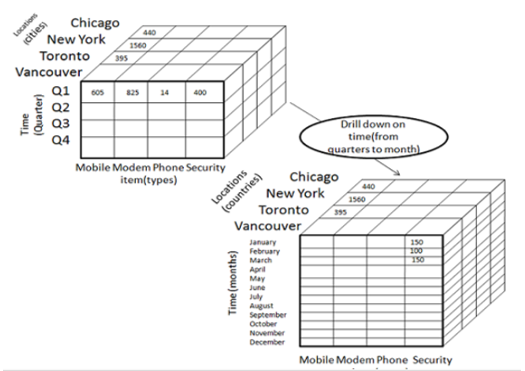Fig 2.2 : Drill Down Operation

### 2.3. OLAP SLICING

The slice operation selects one specific dimension from a given cube and provides a replacement sub-cube.

Slice is that the act of selecting an oblong set of a cube by selecting one worth for one in all its dimensions, making a replacement cube with one fewer dimension.

The picture shows a slicing operation.

Slicing is performed for the dimension "time" using the criteria time = "Q1".

Subcube is formed by using or selecting one or two dimensions.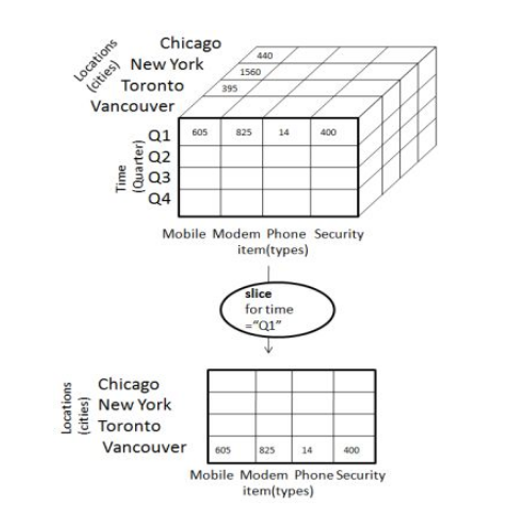Fig 2.3 : OLAP Slicing

### 2.4. OLAP DICING

Dice selects 2 or additional dimensions from a given cube and provides a brand new sub-cube. think about the subsequent diagram that shows the dice operation. The dice operation produces a subcube by permitting the analyst to choose specific values of multiple dimensions. the image shows a dicing operation. The dicing operation on the cube which involves three dimensions is based on the following selection criteria,

• (location = "Toronto" or "Vancouver")
• (time = "Q1" or "Q2")
• (item =" Mobile" or "Modem")Fig 2.4 : Cube Dicing

### 2.5. OLAP PIVOT

The pivot operation is additionally referred to as rotation. It rotates the info axes seeable to produce an alternate presentation of knowledge. take into account the subsequent diagram that shows the pivot operation. Pivot permits AN analyst to rotate the cube in the area to check its varied faces. As an example, cities may well be organized vertically and product horizontally whereas viewing information for a selected quarter. Pivoting may replace the product with periods to check information across time for one product. The picture shows a pivoting operation: the full cube is turned, giving another perspective on the data.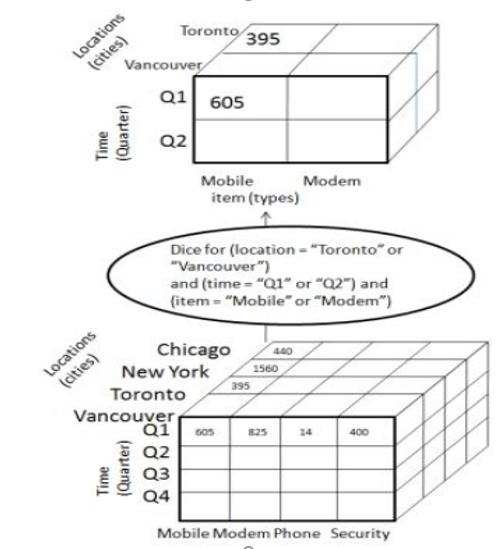Fig 2.5 : Olap Pivot